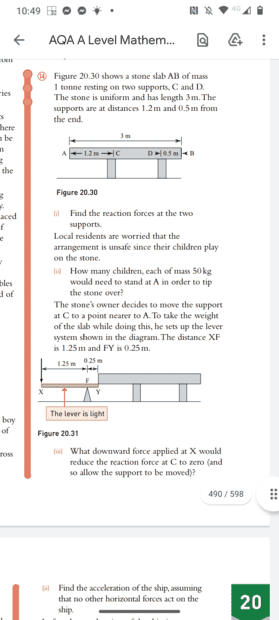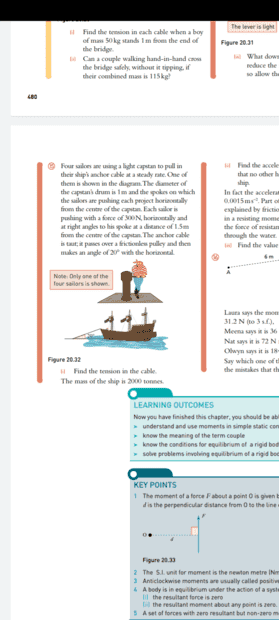# Help with moments questions -- Stone slab resting on two supports

danjsmith98
Homework Statement:
Hi I am stuck on part ii of this question i keep ending up with equations with three unknown variables and don't know how to solve it, any help would be massively appreciated!
Thanks
Relevant Equations:
F=mdHomework Helper
Gold Member
What is your attempt at a solution, for instance what are the three variables you think you have to find?

danjsmith98
So far I have
50n×1.2 +1.3×Rd =1000g×0.3
50n×2.5 + 1000g×1= Rc×1.3

Homework Helper
Gold Member
Welcome, danjsmith98!

Shouldn't 50 kg (mass of each child) be affected by g in order to become a force to be considered in the equations of balance of moments?

Shouldn't the vertical reaction force from support D approach a value of zero when the weight of the children is inducing the stone to tip over?

danjsmith98
Cant believe I didn't times the 50 by g 🤦‍♂️ Thank you so much I've been stressing about this question all day you're a lifesaver!

•Lnewqban
Homework Helper
Gold Member
50n×1.2 +1.3×Rd =1000g×0.3
1. What can you say about the value of Rd when the stone is about to tip?
2. 50n×1.2 is a mass x a distance, 1000gx0.3 is a mass x g x a distance (and 1.3xRd is a force x a distance but bearing in mind the answer to my previous question...): does this look right?

danjsmith98
Thank you mate you've been really helpful I just got the right answer and have been stressing about it all day!
Just wondering if you could help with this next question. I have done 300 x 1.5 = 450, 450 x 4 = 1800
but the answer in the book is 3600, I am not sure if this is due to couples? as i don't really understand themHomework Helper
Gold Member
You can see that your answer is wrong - always show your units: 300N x 1.5m x 4 = 1,800Nm, does this look like a tension? This could have helped you with your first question too.

You can solve this either with couples or with torques; which do you feel more comfortable with?

•Lnewqban
danjsmith98
Oh ok i never really show my units so will start doing it now thanks. Definitely couples we haven't learned about torque just yet

Homework Helper
Gold Member
Well there's not really much difference anywaySo you have correctly calculated that the four sailors exert a force of 1,200N at a distance 1.5m from the centre of the capstan (this is a torque of 1,800Nm). You have been provided with the diameter of the capstan drum, what difference do you think that makes to the tension in the cable?

danjsmith98
Well there's not really much difference anywaySo you have correctly calculated that the four sailors exert a force of 1,200N at a distance 1.5m from the centre of the capstan (this is a torque of 1,800Nm). You have been provided with the diameter of the capstan drum, what difference do you think that makes to the tension in the cable?
Im not too sure, I don't really see what effect it would have on it, from the answer I am guessing it doubles the force but don't know how they got to that conclusion

Homework Helper
Gold Member
You used the distance of 1.5m in your calculation of the effect of the sailors force, how did you get to that conclusion?

danjsmith98
Because the moment about a point is the perpendicular force times the distance to that point and its 1.5m from the sailor to the centre.

Homework Helper
Gold Member
Yes! So if T is the tension in the anchor cable and it is on a drum with a diameter of 1m, what is it's moment about the centre of the drum?

danjsmith98
I would assume from the numbers 0.5 T=F as R=0.5 which equals the distance from the cable to the centre but I would have thought that T=0.5 F not the other way round

Homework Helper
Gold Member
I would assume from the numbers 0.5 T=F as R=0.5 which equals the distance from the cable to the centre but I would have thought that T=0.5 F not the other way round
Don't use F for a moment, that will confuse everyone!

Force x Distance = Moment
Sailors: Force 1,200N x Distance 1.5m = Moment 1,800Nm
Cable: Force (Tension) T x Distance 0.5m = Moment 1,800Nm (it must be equal and opposite to the sailor's moment, ingnoring friction, yes?)

danjsmith98
Ohh ok i see I didnt realize the forces were balancing out but looking back through the question I can see now
that the moments have to be equal thank you so much you've been a massive help!that the moments have to be equal thank you so much you've been a massive help!•